Courses

# Test: Covalent Bonding

## 19 Questions MCQ Test Chemistry for JEE | Test: Covalent Bonding

Description
This mock test of Test: Covalent Bonding for JEE helps you for every JEE entrance exam. This contains 19 Multiple Choice Questions for JEE Test: Covalent Bonding (mcq) to study with solutions a complete question bank. The solved questions answers in this Test: Covalent Bonding quiz give you a good mix of easy questions and tough questions. JEE students definitely take this Test: Covalent Bonding exercise for a better result in the exam. You can find other Test: Covalent Bonding extra questions, long questions & short questions for JEE on EduRev as well by searching above.
QUESTION: 1

### Direction (Q. Nos. 1-16) This section contains 16 multiple choice questions. Each question has four choices (a), (b), (c) and (d), out of which ONLY ONE option is correct. Q. Octet rule is not followed in

Solution: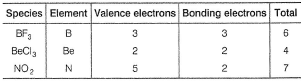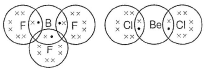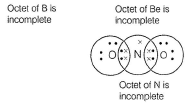QUESTION: 2

### Select the correct statement about valence bond approach.

Solution:

(a) Hs maximum overlap - correct.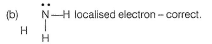(c) Based on valence bond approach,-no prediction of change of structure due to (Ip-Ip), (Ip-bp) repulsions- correct.

QUESTION: 3

### In which of the following substances will hydrogen bond be strongest?

Solution:

HC1, HI and H2S do not from H-bonds. Only H2O forms hydrogen bonds. One H2O molecule forms four H-bonding.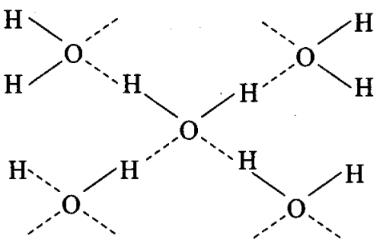QUESTION: 4

The common features among the species CN-, CO and NO+ are

Solution:

According to M.O theory,

CN,CO and NO+ are isoelectronic (14e) and bond order is 3.

Hence (a) is the correct answer.

QUESTION: 5

The correct order of increasing bond length of
I. C— H
II. C— O
III. C— C
IV. C=C

Solution:

H is smallest in size thus, (C— H)bond length is least.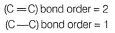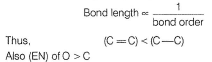Hence, bonding pair in (C— O) is nearer to nucleus decreasing bond length as compared to (C— C).
Thus, I < IV < II < III.

QUESTION: 6

Ratio of σ and π-bonds is maximum in

Solution: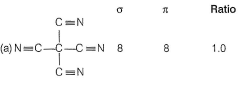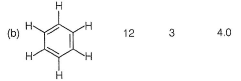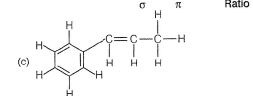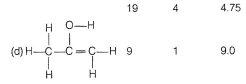QUESTION: 7

In which of the following cases, covalent bonds are cleaved?

Solution:

(a) Boiling of H2O Intermolecular H-bonds are cleaved.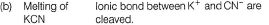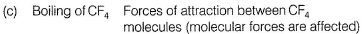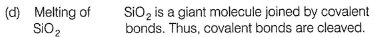QUESTION: 8

The compound (NH3, BF3) can be easily separated into its individual components because

Solution: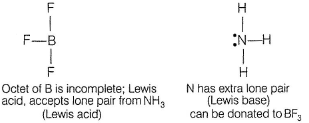Thus, in (NH3,BF3), both have independent existence and thus, it can be separated.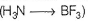QUESTION: 9

In allene (C3H4), the type(s) of hybridisation of the carbon atoms is (are)

Solution:

In allene, hybridization of C-atoms:

The two C-atoms H2-C=C=C-H2 at terminal have sp2 while the middle have sp .

QUESTION: 10

The bond between two identical nonmetal atoms has a pair of electrons:

Solution:

When bond is formed between identical atoms, it is a perfectly covalent bond. Electrons will be shared equally among them.

QUESTION: 11

Following is the dot structure of XY4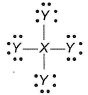Q. X and Y can be

Solution:

There are three lone pairs on Y and four σ - bonds between X and Y.
Thus, octets of X and Y are complete.
X has four electrons in valence shell.
Y has seven electrons in valence shell.
Thus, X = Si (group 14), y = Cl (group 17)

QUESTION: 12

Hyperconjugation involve overlap of the following orbitals

Solution:

Hyperconjugation takes place in allylic compounds in which alkyl group (having at least one H) is attached to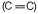bond .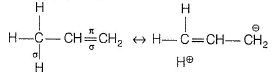σ-bond of (— CH3) and π -bond of (ene) are involved, π-bond is formed due to overlapping of p-orbitals. Thus, σ-orbital and p-orbital are involved.

QUESTION: 13

Malleability and ductility of metals can be accounted due to

Solution:

In metallic bonds, the valence shell electrons are delocalised and shared between many atoms. These delocalised electrons allow the metal atoms to slide past one another without being subjected to strong repulsive forces. The malleability and ductility of metals is due to this sliding capacity of the delocalised electrons.

QUESTION: 14

Polarity in a molecule and hence the dipole moment depends primarily on electronegativity of the constituent atoms and shape of a molecule. Which of the following has the highest dipole moment ?

Solution:

H2O will have highest dipole moment due to maximum difference in electronegativity of H and O atoms.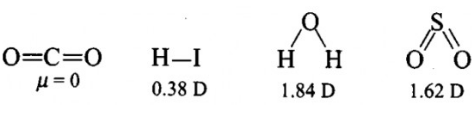QUESTION: 15

Which of the following is nonpolar?

Solution: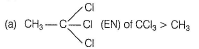(b) PCI5 triangular bipyramidal, symmetrical, no lone pair.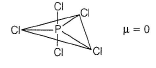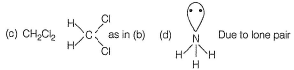QUESTION: 16

The bond between B and C will be

Solution:

Both B and C are non-metals and therefore, bond formed between them will be covalent.

QUESTION: 17

Direction (Q. Nos. 17) Choice the correct combination of elements and column I and coloumn II  are given as option (a), (b), (c) and (d), out of which ONE option is correct.

Q.
Match the orbital overlap figures shown in Column I with the description given in Column II and select the correct answer using the code given below the columns.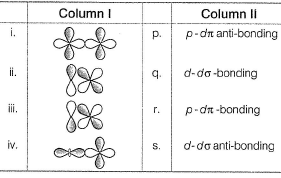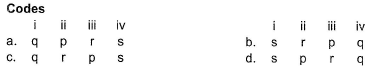Solution:

(i) (d-d) σ-bonding (ii) (p-dπ bonding)
(iii) (p-dπ anti-bonding) (iv) (ddσ anti-bonding)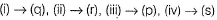QUESTION: 18

Direction (Q. Nos. 18-19) This section contains 2 multiple choice questions. Each question has four choices (a), (b), (c) and (d), out of which ONE or  MORE Then ONE  is correct.

Peroxide ion ______.

a) is diamagnetic.
b) has five completely filled antibonding molecular orbitals.
c) is isoelectronic with neon.
d) has bond order one.

Which one of these is correct?

Solution: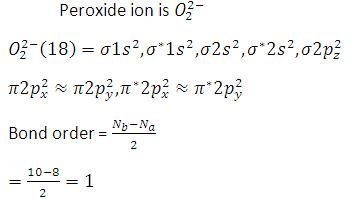It contains four completely filled antibonding molecular orbitals. Since, all the electrons are paired,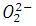is diamagnetic. Peroxide ion is isoelectronic with argon, not with neon.

*Multiple options can be correct
QUESTION: 19

Select the correct statement(s) about divalent anion of orthophosphoric acid.

Solution:

It is a base because it presents three OH group and bond order of PO is equal to total no. of sigma and pie bond/total no of resonance structure due to which = 5/4 = 1.25 ~1.3